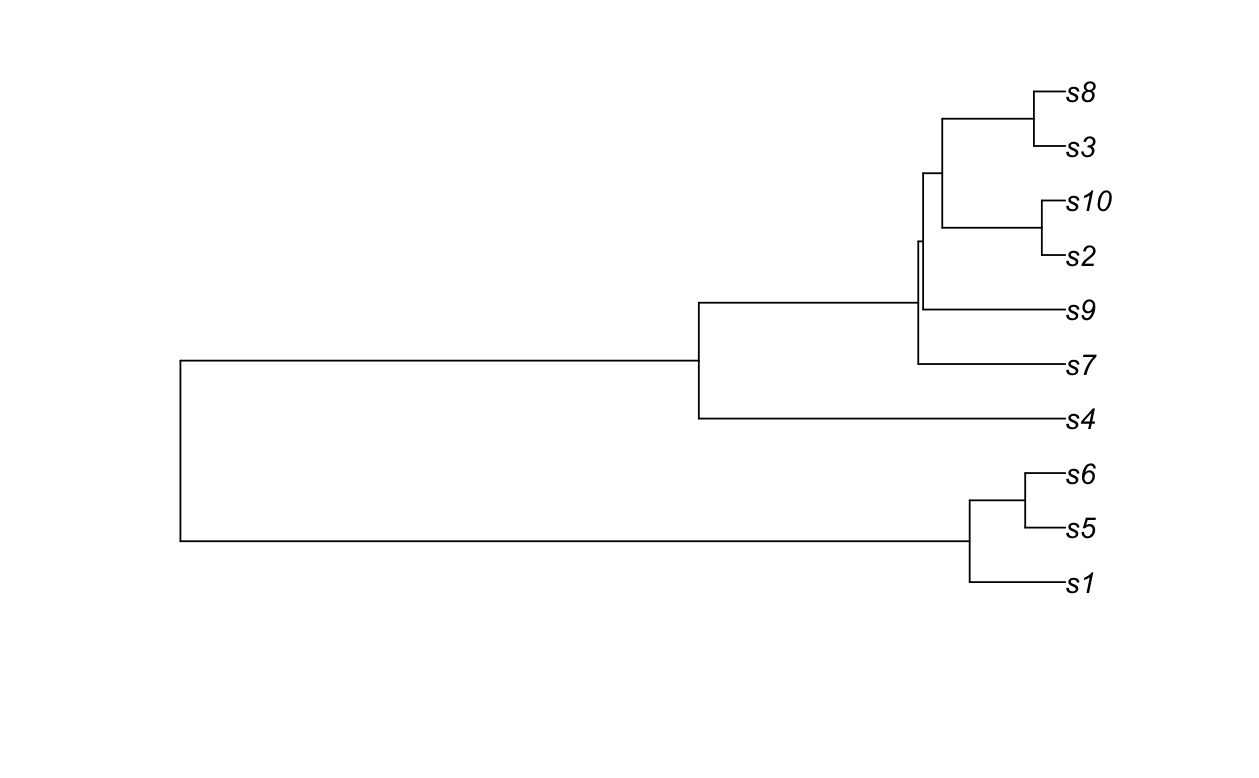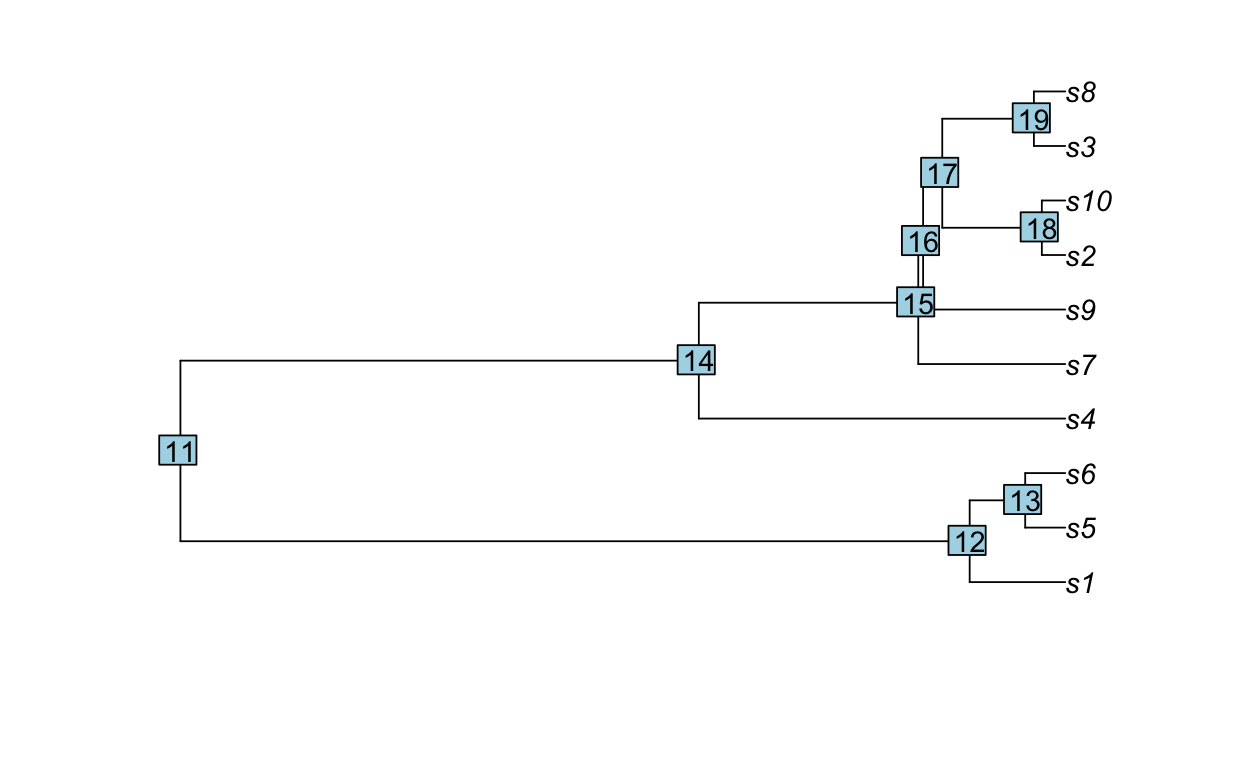We use `ms()` from `phyclust` to simulate samples from the coalescent and various functions from `ape` to display the coalescent tree and calculate statistics from it. Let’s start with a really simple example of constructing a coalescent sample of size 10. The `nsam` argument is the number of samples, and the `opts = "-T"` argument tell `ms()` to return a coalescent tree. We then convert it, using `read.tree()` to a format that we can `plot()`

## The coalescent in `R`

``````library(ape)
library(phyclust)

rm(list = ls())

ms_out <- ms(nsam = 10, opts = "-T")
plot(ms_tree_1)``````You’ll notice that the tip labels run from `s1` through `s25`. Each corresponds to a different allele. That was pretty easy, but we can do a bit more. We can ask how “deep” the tree is, i.e., how far back in time we have to go to find the most recent common ancestor of all the alleles in our sample.

### Branching times

``branching.times(ms_tree_1)``
``````       11
0.7630929 ``````

You’re probably wondering about the `` after `branching.times()`. Well, `branching.times()` returns a vector of all of the branching times in the tree.

``branching.times(ms_tree_1)``
``````        11         12         13         14         15         16
0.76309287 0.08241319 0.03451869 0.31600639 0.12675099 0.12256396
17         18         19
0.10605252 0.02017499 0.02702544 ``````

The numbers 11-19 are the node numbers in the tree above.

``````plot(ms_tree_1)
nodelabels()``````As you can see, node 11 (which is the first one in the list), is the deepest node, i.e., the most recent common ancestor of all of the alleles in our sample.

### Scaling of branching times

The other thing you may be wondering is how to interpret the number 0.7630929 that corresponds to the time of the most recent common ancestor.1 To explain that I have to point out something that you may have missed when we called `ms()`: We didn’t specify a population size. How can we get away with that, since I told you that drift always depends on the population size?

Well, remember what you know about the coalesenct process. The expected time to coalescence of all alleles is \(4N_e\). `ms()` reports branching time in “coalescent units” where 1 unit equals \(4N_e\). So if `branching.times(ms_tree_1)` is 0.7630929 and the effective size of our population is 25, then the time (number of generations before the present) to the most recent common ancestor is 76.3092874. Notice that the time isn’t an integer the way you’d expect it to be. That’s because of an approximation that is generally used in implementing the coalescent in software. If you want to turn it into an integer, you can do this:

``round(4*25*branching.times(ms_tree_1))``
``````11
76 ``````

Whether you round it or not, you’ll see that the most recent common ancestor of the alleles in our sample didn’t occur exactly 100 generations ago as we’d expect. If, however, we repeat that simulation a bunch of times, you can see that we come pretty close - on average.

``````library(ggplot2)

N_e = 25

c_time <- numeric(0)
for (i in 1:1000) {
ms_out <- ms(nsam = N_e, opts = "-T")